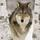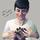## General Question# Can someone help me out with my math homework?

Asked by bluejay (1014) October 18th, 2011

I know how to get the slope of a line when I’m given 2 coordinate pairs, but I don’t know how to do it with only one ordered pair and the y-intercept. Can someone explain it to me or link me to a website? Also how can I find the y-intercept when I have 2 coordinate pairs?

Observing members: 0Composing members: 0Sure. Here on Fluther, we’re not allowed to tell you exactly how to do a homework problem, but I can point you in the right direction.

The slope-intercept formula for a line is y=mx+b, where b is the y coordinate of the y intercept, and m is the slope. The formula for slope is m = (y1-y2)/(x1-x2) where (x1, y1) and (x2, y2) are any two points on the line. Can you figure it out now? I can help you more if you’re still confused.

Also, this shouldn’t vary from what you’re used to. If you’re given one ordered pair and the y intercept, you still have two points. Hint: The x coordinate of the y intercept is always 0.

Mariah (25883)“Great Answer” (8) Flag as…Oh, and for your second question. You said you know how to get the formula for a line when you have two coordinate pairs, right? What form is that formula in? If it’s already in the slope-intercept form (y=mx+b), the intercept is included right in the formula: (0,b). If you’re using another form, I can still help you out, but just let me know what form you’re using first.

Mariah (25883)“Great Answer” (7) Flag as…Thank you!!! (lurved)

bluejay (1014)“Great Answer” (2) Flag as…or